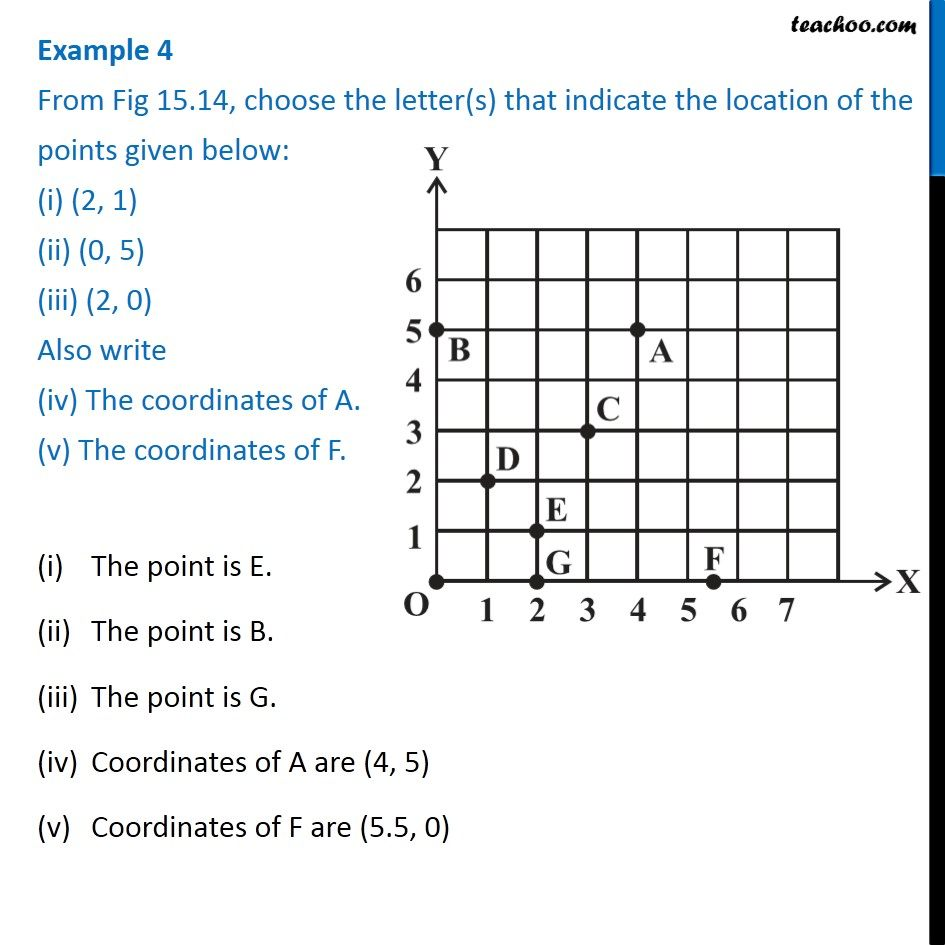Examples

Chapter 15 Class 8 Introduction to Graphs
Serial order wise### Transcript

Example 4 From Fig 15.14, choose the letter(s) that indicate the location of the points given below: (i) (2, 1) (ii) (0, 5) (iii) (2, 0) Also write (iv) The coordinates of A. (v) The coordinates of F. The point is E. The point is B. The point is G. Coordinates of A are (4, 5) Coordinates of F are (5.5, 0)